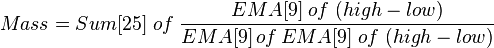# Mass index

The mass index is an indicator, developed by Donald Dorsey, used in technical analysis to predict trend reversals. It is based on the notion that there is a tendency for reversal when the price range widens, and therefore compares previous trading ranges (highs minus lows).

Mass index for a commodity is obtained by calculating its exponential moving average over a 9-day period and the exponential moving average of this average (a "double" average), and summing the ratio of these two over a given amount of days (usually 25).$Mass = Sum \; of \; { EMA \; of \; (high-low) \over EMA\,of\,EMA \; of \; (high-low) }$

Generally the EMA and the re-smoothed EMA of EMA are fairly close, making their ratio is roughly 1 and the sum around 25.

According to Dorsey, a so-called "reversal bulge" is a probable signal of trend reversal (regardless of the trend's direction). Such a bulge takes place when a 25-day mass index reaches 27.0 and then falls to below 26 (or 26.5). A 9-day prime moving average is usually used to determine whether the bulge is a buy or sell signal.

This formula uses intraday range values: not the "true range," which adjusts for full and partial gaps. Also, the "bulge" does not indicate direction.

## References

1. Mass Index construction at IncredibleCharts.com
2. Mass Index at IncredibleCharts.com